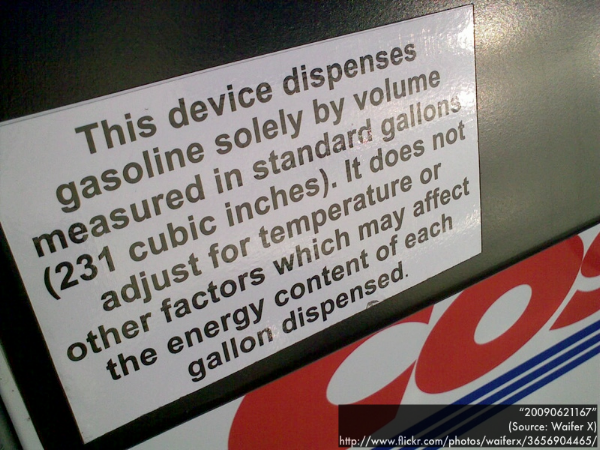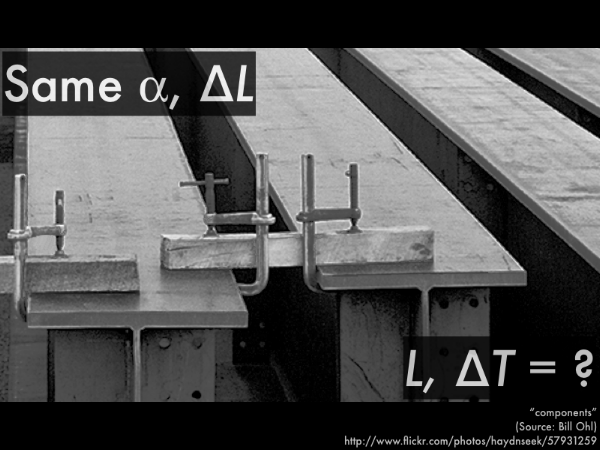## 20141116

### Physics presentation: temperatureWhen is the best time to pull into a gas station to fill up your tank? During the night, when the temperature is cooler, or during the day, when the temperature is warmest? Because the volume of gasoline contracts or expands depending on the temperature.Most fuel companies compensate for these temperature-dependent volume changes, but some do not, and notify you with a sticker notification. If a fuel company does not compensate for temperature, are you necessarily getting ripped off? Or can this be used to your advantage?So let's consider the connection between temperature and the linear and volume expansion of solids and liquids. In fact, the expansion of materials is what ultimately defines what a change in temperature "is."Start with a working definition of temperature and temperature changes (a more precise definition will follow in a subsequent presentation). Hot things have high temperatures, cool things have low temperatures. An increase or decrease in temperature then corresponds to an object heating up, or cooling off. For the purposes of this course we'll use Celsius (° C) and kelvin (K) scales, so don't worry about having to convert to and from Fahrenheit. Note that while the Celsius and kelvin temperature scales are offset from each other, the change in temperature ∆T will be the same whether expressed in Celsius or kelvin.The key to length and volume expansion and contraction is not the temperature, but the changes (increases/decreases) in temperature. This is extremely important when constructing structures, as gaps are typically designed to account for the changes in size depending on temperature.First, linear expansion.We've already discussed how stress causes strain for elastic solids. Here instead of applying force to an object to change its length, we'll apply thermal stress by changing the temperature of an object to change its length. The material-dependent linear expansion coefficient (in units of inverse kelvin, or alternatively inverse Celsius) characterizes the response of the material to thermal stress. As a result, both sides of this relation are unitless.These steel beams have the same expansion coefficient α (being made of the same material). Assume that we can heat them separately in order to get them to each expand by 1.0 cm from their original lengths.

For the different length steel beams, which will require a greater temperature increase to expand by 1.0 cm from its original length: the shorter beam, or the longer beam?

Let's rewrite the linear expansion equation in terms of L and ΔT, as these are different for the short and long steel beams, and have the thermal expansion coefficient α and expansion ΔL on the other side of the equation, as these quantities are both the same for both short and long beams:

L⋅ΔT = (∆L/α).

So the right-hand side of this equation is the same for both the short and long beams:

Lshort⋅ΔTshort = (∆L/α),

Llong⋅ΔTlong = (∆L/α),

so we can set the left-hand sides of both these equations equal to each other:

Lshort⋅ΔTshort = Llong⋅ΔTlong.

Since Lshort < Llong, then for the equality to hold, ∆Tshort > ∆Tlong, and thus the shorter beam will require a greater increase in temperature to expand the same amount as the longer beam.Second, extending these linear expansion concepts to volume expansion.Applying thermal stress by changing the temperature of a liquid or solid changes its volume. The material-dependent volume expansion coefficient β (in units of inverse kelvin, or alternatively inverse Celsius) characterizes the response of the material to thermal stress, and for solids this is merely three times the linear expansion coefficient α (due to expansion along each of its three dimensions of length, width, and height). Similar to the linear thermal expansion relation, both sides of this volume expansion relation are unitless.The liquid that fills a container to the brim can undergo an expansion in volume depending on an increase in temperature. However, the liquid may not necessarily overflow this container, as the container will also undergo an expansion in volume with an increase in temperature. Ultimately you would need to compare the volume expansions of both materials--if the liquid expands less than the container's expansion, the level of liquid would be lower; if the liquid expands greater than the container's expansion, then the liquid would overflow. If the liquid and the container both expand the same amount for the same increase in temperature (meaning that their volume expansion coefficients are equal), then the liquid will still fill the container to the brim.This is how thermometers work--the volume of liquid inside the bulb (mercury, or more commonly red-colored alcohol) expands more than the enclosing glass bulb as temperature increases, and the overflow of the red-colored alcohol out of the bulb shows up as a rising level in the attached tube. This is how a given amount of temperature increase was originally intended to be scaled--the temperature difference, say, between freezing and boiling of water would be assigned as a value of "180° (Fahrenheit)" between the corresponding low and high liquid level marks on a thermometer. Then 1/180th of the distance between these two marks would then be assigned a value of a change in temperature of "1° Fahrenheit."

Which material has a greater volume expansion coefficient for this thermometer: glass, or alcohol?This plastic rainwater basin was filled to the brim last night, but ignoring evaporative losses, the next morning, when it was a little cooler, the level of water is lower than the brim of the plastic basin. (For simplicity ignore the unusual slight expansion behavior of water as it cools down to nearly the freezing point, and concentrate on water expanding as it heats up, and contracting as it cools down like most other solids and liquids.)

Which material has a greater volume expansion coefficient: plastic, or water?So back to the gas pumps that do not compensate for temperature. If you fill up your car at a pump that does not adjust for temperature, would it be a better deal to purchase a "cold-dispensed" gallon of gasoline, or a "warm-dispensed" gallon of gasoline--even though the volume of each type of gasoline is exactly one gallon? What is the difference between gasoline dispensed at different temperatures?